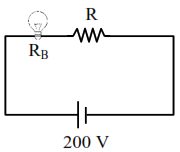# An electric bulb ratedQuestion:

An electric bulb rated as $200 \mathrm{~W}$ at $100 \mathrm{~V}$ is used in a circuit having $200 \mathrm{~V}$ supply. The resistance ' $R$ ' that must be put in series with the bulb so that the bulb delivers the same power is________$\Omega$

Solution:

Power, $\mathrm{P}=\frac{\mathrm{V}^{2}}{\mathrm{R}_{\mathrm{B}}}$

$\mathrm{R}_{\mathrm{B}}=\frac{\mathrm{V}^{2}}{\mathrm{P}}=\frac{100 \times 100}{200}$

$\mathrm{R}_{\mathrm{B}}=50 \Omega$To produce same power, same voltage (i.e. $100 \mathrm{~V}$ ) should be across the bulb

Hence, $R=R_{B}$

$R=50 \Omega$